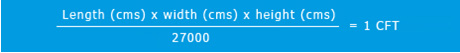﻿ :: Welcome to RCPL ::

# Volumetric Calculator

HomeVolumetric Calculator

Calculation of volumetric weight for determining the cost of shipping the cargo is a standard practice followed throughout the industry. It calculates the space a package will occupy at the time of transit with different modes of transportation and the space occupied in the warehouse. Space occupied is calculated in relation to the weight of the cargo.

Formulas used to calculate the Volumetric Weight are

Measure in Centimeter (cms)Measure in Inches (in)Where 1 CFT = 10 Kg

In order to avoid confusions the measurements of the goods are rounded-off to the nearest whole number

Higher weight of the volumetric and actual weight will be chargeable